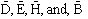EE 333

Electricity & Magnetism

Textbook

Electromagnetic Fields and Waves "Second Edition", Magdy F. Iskander, Waveland Press

Course syllabus

• Review of vector analysis

• Scalar and Vector fields

• Sums and Products of vectors

• Vector integration

• Coordinate systems

• Definition of electric field intensity and magnetic flux density fields in terms of their forces

• Postulation of Maxwell's equations in integral form with validation provided using Gauss's Law, Ampere's Circuital Law, and Faraday's Law

• Solution of problems involving "simple"  static distributions of charge and current using the integral form of Maxwell's equations

• Vector differential relations

• Definition of gradient, divergence, and curl operations

• Definition of the Laplacian operator

• Green's integral theorem: uniqueness

• Graphical proof of Divergence and Stokes Theorems

• Conversion of integral form of Maxwell's equations to differential form

• Plane wave solutions in free space

• Phase velocity

• Polarization

• Intrinsic impedance

• Maxwell's equations in material media

• Effect of finite conductivity

• Effect of electric polarization

• Effect of magnetic polarization

• Development of boundary conditions for• Definition of linear, isotropic, and homogeneous media

• Plane wave solutions in material media

• TEM waves guided by a two conductor transmission line

• Reflection at a lumped parameter load on a transmission line

• General analytical solutions of sinusoidal waves on uniform transmission lines

• Use of the Smith chart for solving transmission line problems

• Transient solutions for lossless transmission lines with an arbitrary load

• Definition of Electrostatic Scalar Potential

• Energy of electrostatic field

• Electrostatic forces and torques